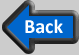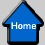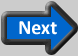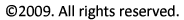Thrust

The mechanism for generating thrust is encompassed by Newtons Third law. That is, propulsion through a fluid is achieved by applying a force to the fluid in one direction, which by Newtons Third law results in a force on the object in the opposite direction. Equivalently, accelerating the fluid in one direction will apply a force (thrust) on the propulsion device in the opposite direction. This is illustrated in the figure below where a propulsion device ingests low velocity fluid and expels high velocity fluid (Ve > V0) to generate a thrust force opposite to the direction of the exhaust fluid motion.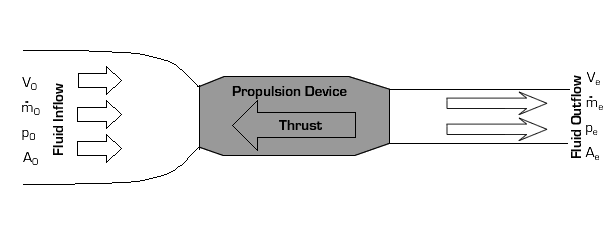As far as Newton’s Third Law is concerned, the exact mechanism by which the propulsion device accelerates the fluid is not relevant. The propulsion device may be a jet engine, a propeller, a flapping fin on a dolphin, etc. The design of the propulsion device does, however, affect the magnitude of the thrust it generates as well as its efficiency, as will be discussed in the Design section.

For a steady-state propulsion device like a jet engine or propeller, thrust can be expressed in terms of the flow parameters as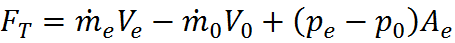where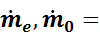the mass flow rate of fluid entering and exiting the propulsion device, respectively,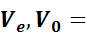the fluid velocity upstream and at the exit of the propulsion device, respectively,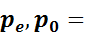the fluid pressure upstream and at the exit of the propulsion device, respectively, and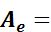the cross-sectional area at the exit of the propulsion device.

Mass flow rate can be expressed as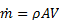where the density (r), area (A), and velocity (V) at the appropriate location are used.

For many cases (such as propellers and subsonic jets), pe = p0. Also, for a steady-state propulsion device, conservation of mass requires that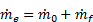where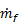is the mass flow rate of fuel into the propulsion device. That is, the rate at which mass exits the propulsion device must equal the rate at which fluid and fuel mass enter the device. Typicallyis small, or even non-existent (for example, a propeller driven by an electric motor), so that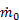. Using these simplifications, the equation for thrust becomes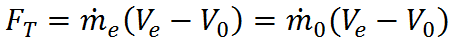Clearly Ve > V0 is required for positive thrust as described earlier (i.e., fluid must be accelerated). Moreover, the larger the difference between Ve and V0 or the larger the mass flow rate of fluid through the propulsion device (), the larger the thrust.

For an unsteady propulsion device, the relationship between the flow parameters and thrust is much more complex, but Newton’s Third Law is still the basic principle governing the resulting propulsive thrust.Example Problem: Engine Comparison